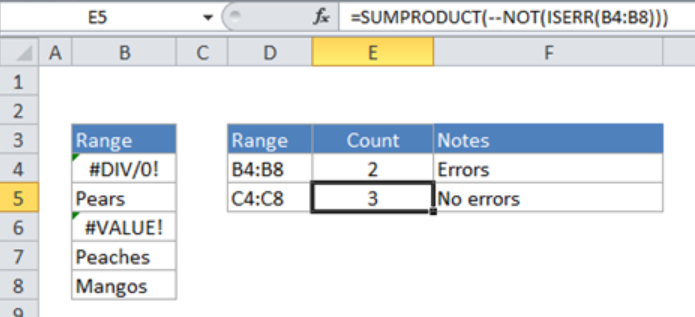Get instant live expert help with Excel or Google Sheets“My Excelchat expert helped me in less than 20 minutes, saving me what would have been 5 hours of work!”

#### Post your problem and you'll get expert help in seconds

Your message must be at least 40 characters
Our professional experts are available now. Your privacy is guaranteed.

# Count Cells That Do Not Contain Errors

As we work on excel spreadsheets, a time comes when we need to count cells that do not contain errors. The NOT function together with ISERR function can be wrapped inside the SUMPRODUCT function to count cells that have errors. This post will step through how to count cells that do not contain errors.Figure 1. Final result

## Syntax of the formula

`=SUMPRODUCT(--NOT(ISERR(rng)))`

Where ‘rng’ represents the range.

## Understanding the formula

The formula has the following parts which play various roles as explained:

SUMPRODUCT: This part will count the total number of products supplied. It accepts single or multiple arrays and will calculate the sum of the products of corresponding numbers. In the event only one array is supplied, then the SUMPRODUCT will just sum the items in that array.

ISERR: this part is evaluated for each cell in the given range. In the absence of NOT, we shall get an array of results equal to TRUE or FALSE, i.e. `{TRUE; FALSE; TRUE; FALSE; FALSE}`

NOT: When this is included in the formula, then we get the result as shown below;

`{FALSE; TRUE; FALSE; TRUE; TRUE}`

The results by the NOT function will correspond to those cells in the range that do not contain errors.

In the formula, we also have the double hyphen, otherwise referred to as double unary. This forces the results (TRUE/FALSE) into 1s and 0s. Thus, `{FALSE; TRUE; FALSE; TRUE; TRUE}` turns to `{0; 1; 0; 1; 1}`

After all these have been done, SUMPRODUCT then sums all the items in the array, and returns the total.

We also have the SUM function which is fundamental in counting errors. The structure os this formula is same as what we have already discussed, with the only difference that it has to be entered as an array formula.

## How to enter an array formula:

Press (Control + Shift + Enter)

This formula looks as below:

`{=SUM(--NOT(ISERR(B4:B8)))}`

Most of the time, the problem you will need to solve will be more complex than a simple application of a formula or function. If you want to save hours of research and frustration, try our live Excelchat service! Our Excel Experts are available 24/7 to answer any Excel question you may have. We guarantee a connection within 30 seconds and a customized solution within 20 minutes.

### Did this post not answer your question? Get a solution from connecting with the expert.Another blog reader asked this question today on Excelchat:## Subscribe to Excelchat.coAnother blog reader asked this question today on Excelchat: